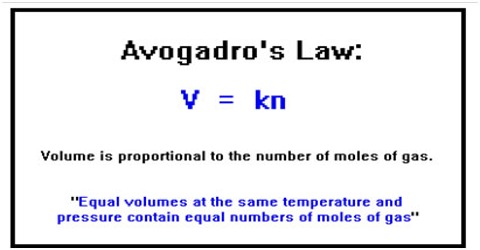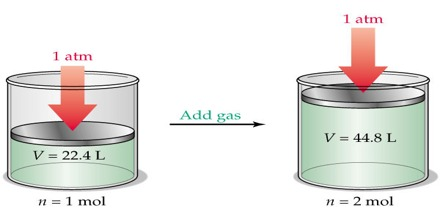# Avogadro’s Law: Explanation in terms of Gaseous State

In describing the behavior of gases Avogadro(1811),  an Italian  physicist proposed that: equal volumes of all gases at the same temperature and pressure contain equal number of molecules.

This is known as Avogadro’s hypothesis or Avogadro’s law.Experimentally it was found that one gram molecular mass (one mole) of some gases at 0°C and 1 atmosphere pressure occupied a volume of 22.414 L. From Avogadro’s law it follows that one gram molecular mass (one mole) of any gas at 0°C and 1 atmosphere pressure (STP. i.e., standard temperature and pressure) will occupy a volume of 22.414 L, since one mole of all gases contains the same number of molecules. Mathematically the law may then be stated as:

V ∞ n [P and T constant)]

Here n is the number of moles of the gas present. The molecular mass expressed in grams is known as the gram molecular mass. One gram mole or one mole signifies that quantity of the substance whose mass is equal to one gram molecular mass.

The volume occupied by one mole of gas is its molar volume and the number of molecules in one mole of a substance is known as the Avogadro number (or Avogadro Constant).